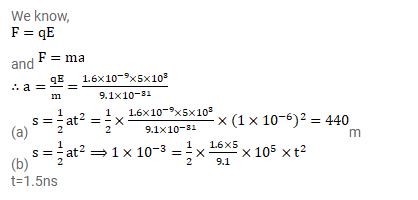# A molecule of a gas, filled in a discharge tube,

Question:

A molecule of a gas, filled in a discharge tube, gets ionized when an electron is detached from it. An electric field of $5.0 \mathrm{kV} / \mathrm{m}$ exists in the vincinity of the event. (a) Find the distance travelled by the free electron in $1 \mu_{\mathrm{S}}$ assuming no collision. (b) If the mean free path of the electron is $1.0 \mathrm{~mm}$, estimate the time of transit of the free electron between successive collisions.

Solution: